Courses

# Test: Number System - 1

## 30 Questions MCQ Test Topic-wise Past Year Questions for CAT | Test: Number System - 1

Description
This mock test of Test: Number System - 1 for CAT helps you for every CAT entrance exam. This contains 30 Multiple Choice Questions for CAT Test: Number System - 1 (mcq) to study with solutions a complete question bank. The solved questions answers in this Test: Number System - 1 quiz give you a good mix of easy questions and tough questions. CAT students definitely take this Test: Number System - 1 exercise for a better result in the exam. You can find other Test: Number System - 1 extra questions, long questions & short questions for CAT on EduRev as well by searching above.
QUESTION: 1

### How many factors of 24 × 35 × 104 are perfect squares which are greater than 1? 

Solution:

24 × 35 × 104 = 28 × 35 × 54
For perfect squares, we have to take only even powers of the prime factors of the number
The number of ways 2’s can be used is 5 i.e. 20, 22, 24, 26, 28
The number of ways 3’s can be used is 3 i.e. 30, 32, 34
The number of ways 5’s can be used is 3 i.e. 50, 52, 54
Therefore, the total number of factors which are perfect squares = 5 × 3 × 3 = 45
But this also includes the number 1.
Hence excluding 1, the required number is 45 – 1 = 44.

QUESTION: 2

### How many pairs (m, n) of positive integers satisfy the equation m2 +105 = n2? 

Solution:

m2 + 105 = n2 ⇒ n2 – m2 = 105
⇒ (n – m) (n + m) = 105
Since m an d n are positive integers (n – m) < (n + m), then by splitting 105 in two factors, we get
⇒ (n – m) (n + m) = 1 × 105
For (n – m) = 1 and (n + m) = 105, (m, n) = (52, 53)
⇒ (n – m) (n + m) =  3 × 35
For (n – m) = 3 and (n + m) = 35 (m, n) = (16, 19)
⇒ (n – m) (n + m) =  5 × 21
For (n – m) = 5 and (n + m) = 21 (m, n) = (8, 13)
⇒ (n – m) (n + m) =  7 × 15
For (n – m) = 7 and (n + m) = 15, (m, n) = (4, 11)
Hence, there are four required pairs.
Shortcut approach :
Number of pairs =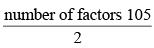105 = 3 × 5 × 7
Factors of 105 are 1, 3, 5, 7, 15, 21, 35 and 105
Number of factors of 105 = 8
Hence, required number of pairs = 8 / 2 = 4

QUESTION: 3

### In a six-digit number, the sixth, that is, the rightmost, digit is the sum of the first three digits, the fifth digit is the sum of first two digits, the third digit is equal to the first digit, the second digit is twice the first digit and the fourth digit is the sum of fifth and sixth digits. Then, the largest possible value of the fourth digit is 

Solution:

Let the number be ABCDEF, where A, B, C, D, E and F be the digits
Now, C =  A, B = 2A
F = A + B + C = A + 2A + A = 4A, E = A + B = A + 2A = 3A
and D =  E + F = 3A + 4A = 7A
Since A an d D both are digit, the maximum possible value of A = 1. Therefore, the maximum value of D = 7

QUESTION: 4

The number of common terms in the two sequences: 15, 19, 23, 27, . . ., 415 and 14, 19, 24, 29, . . ., 464 is



Solution:

Both the given sequences are A.P.
The common difference for the first sequence, d1 = 4
The common difference for the second sequence, d2 = 5
The first common term in the two given A.P. is 19.
The common terms will also be in arithmetic progression with common difference
LCM (d1, d2) = LCM (4, 5) = 20
Let there be ‘n’ terms in this sequence, then the last term would be ≤ 415
Hence for A.P. of common terms
a + (n – 1) d ≤ 415
⇒ 19 + (n – 1) × 20 ≤ 415
⇒ (n – 1) × 20 ≤ 396
⇒ (n – 1) = [396 / 20] where [396 / 20] is the greatest integer
⇒ (n –1) = 19
∴ n = 20

QUESTION: 5

In an examination, the score of A was 10% less than that of B, the score of B was 25% more than that of C, and the score of C was 20% less than that of D. If A scored 72, then the score of D was



Solution:

Let score of A, B, C and D are a, b, c and d respectively.
Score of A = 72,
∴ a = 72
Also a = 0 .9 × b ⇒ b = a/0.9 = 72 / 0.9 = 80
and b = 1 .25 × c ⇒ c = b / 1.25 = 80 / 1.25 = 64
and e = 0.8 × d ⇒ d = c / 0.8 = 64 / 0.8 = 80
Hence score of D = 80

QUESTION: 6

If m and n are integers such that (√2)19 34 42 9m 8n = 3n 16(∜64) then m is



Solution:

(√2)19 34 42 9m 8n = 3n 16(∜64)
⇒ 219/2 × 34 × 24 × 32m × 23n = 3n × 24m × 23/2
⇒ 2(19/2 + 4 + 3n) × 3(4 + 2m) =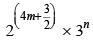On comparing the powers of same bases from both side, we get
19 / 2 + 4 + 3n = 4m + 3 / 2
⇒ 3n = 4m +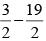- 4
⇒ 3n = 4m +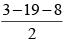⇒ n =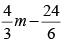⇒ n =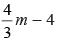...(i)
4 + 2m = n   ...(ii)
From equation (i) and (ii), we get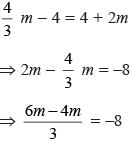⇒ 2m = –24
∴ m = –12

QUESTION: 7

The product of two positive numbers is 616. If the ratio of the difference of their cubes to the cube of their difference is 157 : 3, then the sum of the two numbers is



Solution:

Let the two numbers be x and y
∴ x × y = 616
and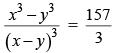Now, let x3 – y3 = 157k and (x – y)3 = 3k
Since, (x – y)3 = x3 – y3 – 3xy(x – y)
∴ 3k = 157k – 3 × 616(3k)1/3
⇒ 154k = 3 × 616 × (3k)1/3 ⇒ k =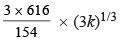⇒ k = 12 × (3k)1/3 ⇒ k3 = 123 × 3 × k
⇒ k(k2 – 123 × 3) = 0
∵ k ≠ 0
∴ k2 = 3 × 123
∴ k = 72
⇒ x – y = (3k)1/3 = (3 × 72)1/3 = 6
∵ (x + y)2 = (x – y)2 + 4xy
∴ (x + y)2 = 62 + 4 × 616 = 2500
⇒ x + y = 50
Hence, sum of the two numbers = 50

QUESTION: 8

If (5.55)x = (0.555)y = 1000, then the value of 1/x - 1/y is



Solution:

Given, (5.55)x = 1000 ⇒ (5.55)x = 103
Taking log both the sides, we get
x log10(5.55) = 3 ⇒ log10(5.55) = 3 / x
⇒ log10(10 × 0.555) = 3 / x
⇒ log10(0.555) + 1 = 3 / x  ...(i)
Also given, (0.555)y = 1000
Taking log both the sides, we get
y log10(0.555) = 3 ⇒ log10(0.555) = 3 / y  ...(ii)
From (i) and (ii), we get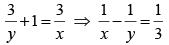QUESTION: 9

The number of integers x such that 0.25 < 2x < 200, and 2x + 2 is perfectly divisible by either 3 or 4, is



Solution:

0.25 < 2x < 200
Possible values of x satisfying the above inequality are –2, –1, 0, 1, 2, 3, 4, 5, 6, 7. When x = 0, 1, 2, 4 and 6, 2x + 2 is divisible by 3 or 4.
Hence, required number of values of x is 5.

QUESTION: 10

While multiplying three real numbers, Ashok took one of the numbers as 73 instead of 37. As a result, the product went up by 720. Then the minimum possible value of the sum of squares of the other two numbers is



Solution:

Let the other two numbers be y and z.
73yz – 37yz = 720
yz = 20
Minimum possible sum of the squares of the other two numbers would occur when y = z
If y = z, then y2 = 20 = z2
Hence, y2 + z2 = 40

QUESTION: 11

The number of girls appearing for an admission test is twice the number of boys. If 30% of the girls and 45% of the boys get admission, the percentage of candidates who do not get admission is



Solution:

Let the number of girls be 2x and number of boys be x.
Girls getting admission = 0.6 x
Boys getting admission = 0.45 x
Number of students not getting admission = 3x – 0.6x – 0.45x = 1.95x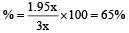Alternatively,
Let the no. of boys appearing for the admission test be b % of candidates who get admission.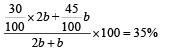QUESTION: 12

A natural number n is such that 120 ≤ n ≤ 240. If HCF of n and 240 is 1, how many values of n are possible?

(2016)

Solution:

Here, 120 ≤ n ≤ 240.
120 = 23 (3)(5) and 240 = 24 (3)(5)
So, the prime factors involved in 120 and 240 are the same.
So, number of co-primes of 240 lying between 120 and 240 = φ(240) – φ(120).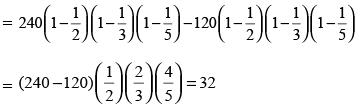QUESTION: 13

If N = 888…up to 100 digits, what is the remainder when N is divided by 625?

(2016)

Solution:

Remainder [N/625]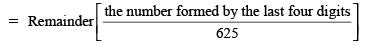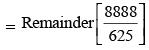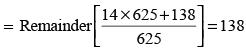QUESTION: 14

A sequence of 4 digits, when considered as a number in base 10 is four times the number it represents in base 6. What is the sum of the digits of the sequence?

(2016)

Solution:

Let the 4-digit sequence be abcd.
In base 6, this represents 216a + 36b + 6c + d and each of a, b, c, d is less than 6.
In base 10, it represents 1000a + 100b + 10c + d.
Given 4(216a + 36b + 6c + d)
= 1000a + 100b + 10c + d
⇒ 136a = 44b + 14c + 3d ...(A)
By trial a = 1, b = 2, c = 3, d = 2
If a = 2, the LHS = 272
[If we consider b = 5, we need 272 – 220 or 52 from 14c + 3d (c, d)=(2, 8) but 8 is not a proper digit in base 6.
If a= 3, the LHS = 408, while 44b + 14c + 3d can at the most be (44 + 14 + 3) 5 or 305.
∴ There are no other possible values that satisfy (A)]
∴ abcd = 1232 and a + b + c + d = 8

QUESTION: 15

What is the remainder when 7700 is divided by 100?

(2016)

Solution:

Here, 74 = 2401
∴ 7700 = (74)175 = (2401)175
Any power of 2401 will end with 1 as the units digit and 0 as the tens digit.
∴ When it is divided by 100, the remainder is 1.

QUESTION: 16

How many ordered triplets (a, b, c) exist such that LCM (a, b) = 1000, LCM (b, c) = 2000, LCM (c, a) = 2000 and HCF (a, b) = k × 125?

(2015)

Solution:

1000 = 23 × 53 and 2000 = 24 × 53
Since LCM (c, a) and LCM (b, c) is 24 × 53 and LCM (a, b) = 23 × 53, so the factor 24 must be present in c.
Hence c = 24 × 5x, where x ranges from 0 to 3 Therefore, there are four possible values of C.
Since, HCF of (a, b) = K × 53, it means
a = 2y × 53
b = 2z × 53
x = 0 to 3, y = 0, then z = 3 → 4 cases.
x = 0 to 3, y = 1, then z = 3 → 4 cases.
x = 0 to 3, y = 2, then z = 3 → 4 cases.
x = 0 to 3, y = 3, then z = 3 → 4 cases.
x = 0 to 3, y = 3, then z = 2 → 4 cases.
x = 0 to 3, y = 3, then z = 1 → 4 cases.
x = 0 to 3, y = 3, then z = 0 → 4 cases.
Hence, total cases = 28.

QUESTION: 17

Which of the following will completely divide (10690 – 4990)?

(2015)

Solution:

x = 10690 – 4990
∵ (xn – an) is divisible by both (x – a) and (x + a) whenever n is even
⇒ (10690 – 4990) is divisible by both 57 and 155
57 = 19 × 3
155 = 31 × 5
Therefore, (10690 – 4990) will be divisible by (19 × 31) = 589 as well.
Also, note that (10690– 4990) will be odd and options (b) and (c) are even. Hence, they can be rejected.

QUESTION: 18

Let P be the set of all odd positive integers such that every element in P satisfies the following conditions.
I. 100 ≤ n < 1000
II. The digit at the hundred’s place is never greater than the digit at tens place and also never less than the digit at units place.

How many elements are there in P?

(2015)

Solution:

Let n be xyz and since n is odd z can take only odd values i.e. 1, 3, 5 and 9 Now, x ≤ y and x ≥ z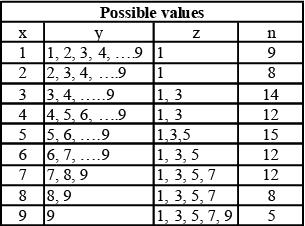∴ Total number of elemenls in P = 95.

QUESTION: 19

A four-digit number is divisible by the sum of its digits. Also, the sum of these four digits equals the product of the digits. What could be the product of the digits of such a number?

(2015)

Solution:

Solve by option.
Option (a): If the product of the digits is 6. then the factors of 6 are 1,2, 3 and 6. This combination of digits is not suitable. So it is not the answer.
Option (b): If the product of the digits is 8, then the factors of 8 are 1, 2, 4 and 8. So only possible combination is 1, 1, 2, 4.
Hence, the number is 4112. It is suitable for answer.
Similarly, we can check options (c) and (d).

QUESTION: 20

P is the product of the first 100 multiples of 15 and Q is the product of the first 50 multiples of 2520. Find the number of consecutive zeroes at the end of P2/Q × 101767

(2015)

Solution:

P = 15100 (1 × 2 × 3 × .... × 100)
= 15100 × 100!
Highest power of 2 in P = 97 (2 will be deciding factor for number of zeroes because number of lives will be greater than number of zeroes in this number) Q = 2520×50 (1 × 2 × 3x .... × 50) = 52000 × 50!
Highest power of 2 in θ = 47
So Highest power of 2 in
P2/Q × 101767 = 2 × 97 + 1767 – 47 = 1914
Hence, number of zeroes = 1914.

QUESTION: 21

x is the smallest positive integer such that when it is divided by 7, 8 and 9 leaves remainder as 4, 5 and 6 respectively. Find the remainder when x3 + 2x2 – x – 3 is divided by 132.

(2015)

Solution:

x = L.C.M. of (7, 8, 9) – 3 = 504 – 3 = 501
x3 + 2x2 + x – 3 = (x – 1) (x + 1) (x + 2) –1
= 500 × 502 × 503 – 1
Remainder when 500 × 502 × 503 – 1 is divided by:
11 = 4
3 = 0
4 = 3
Required remainder = least possible number which when divided by 11, 3 and 4 leavs remainder 4, 0 and 3 respectively.
Such least no. is 15.

QUESTION: 22

‘P’ is the product of ten consecutive two-digit natural numbers. If 2a is one of the factors of P, then the maximum value that ‘a’ can assume is

(2014)

Solution:

In order to maximize the power of 2 in the product, one of the ten numbers has to be 64 as this is the highest two–digit number of the form 2k, where k is a natural number.
There has to be maximum number of multiples of 8 among the ten numbers. In a set of ten consecutive natural numbers, there can be a maximum of two numbers that will be a multiple of 8.
The possible sets of ten consecutive natural numbers that satisfy the aforementioned conditions are 55 to 64, 56 to 65, 63 to 72 and 64 to 73. The highest power of 2 in the product of any of these sets of ten numbers will be 13.

QUESTION: 23

If 7a = 26 and 343b = 676 then what is the relation between a and b?

(2014)

Solution:

Here, 343b = 676
⇒ 73b = 262
Now,7a = 26
⇒ 73b = (7a)2
⇒ 2a = 3b

QUESTION: 24

A set ‘P’ is formed from the set of first ‘N’ natural numbers by deleting all the perfect squares and all the perfect cubes. If the numbers are arranged in an ascending order then, what is the 476th number of the set ‘P’?

(2014)

Solution:

Here, we take the 1st option, a delete all perfect squares and perfect cubes, then a total of 22 perfect square will be deleted (12, 22 ........ 222) and a total of 7 perfect cubes will be deleted (13 23,.... 73) and Two numbers are common in between them viz. 16 and 26 which are perfect squares as well as perfect cubes Thus, 500 is the (500 – 22 – 7 + 2) = 473 rd term.
So, 476th term = 500 + 3 = 503.

QUESTION: 25

Find the number of ways in which a batsman can score 100 runs by scoring runs in 2’s, 4’s and 6’s, such that he hits at least one double, one boundary and one six.

(2014)

Solution:

Let the batsman scored a 2's, b 4's and c 6's.
⇒ 2a + 4b + 6c = 100
⇒ a + 2b + 3c = 50. ...(i)
When c = 1, (i) becomes a + 2b = 47
⇒ a = 47 – 2b ....(ii)
Since a ≥ 1 and b ≥ 1, the number of solutions of (ii) is 23.
When c = 2, (i) becomes a + 2b = 44
⇒ a = 44 – 2b ...(iii)
Since a ≥ 1 and b ≥ 1, the number of solutions of (iii) is 21.
When c = 3, (i) becomes a + 2b = 41
⇒ a = 41 – 2b ...(iv)
Since a ≥ 1 and b ≥ 1, the number of solutions of (iv) is 20.
When c = 4, (i) becomes a + 2b = 38
⇒  a = 38 –2b ...(v)
Since a ≥ 1 and b ≥ 1, the number of solutions of (v) is 18.
Thus, we see a pattern emerging.
∴ The total number of ways
= 23 + 21 + 20 + 18 + ... + 3 + 2 = 184.

QUESTION: 26

The sequence P1, P2, P3, ..... is defined by P1 = 211, P2 = 375, P3 = 420, P4 = 523, and Pn = Pn-1 – Pn- 2 + Pn - 3 – Pn - 4 for all n3 5. What will be the value of P531 + P753 + P975?

(2014)

Solution:

Put n = 5, in the given relation then, P5 = 267 Again,
P6 = P5 – P4 + P3 – P2
⇒ P6 = – P1
P7 = – P2,
Similarly, P8 = – P3
P9 = – P4
P10 = – P5
The sequence repeats its terms after every 10 terms.
Here, we observe following pattern
P531 = P(530 + 1) = P1 = 211
P753 = P(750 + 3) = P3 = 420
P975 = P(970 + 5) = P5 = 267
So, P531 + P753 + P975 = 211 + 420 + 267 = 898.

QUESTION: 27

The ratio of two numbers whose sum is 600 is 7 : 8. What is the LCM of the given two numbers?

(2014)

Solution:

Let the two numbers be x and y according to question,
x + y = 600 and x/y = 7/8
∵ x + y = 600 ...(i)
x/y = 7/8 ⇒ 8x - 7y = 0 .....(ii)
From equation (i) and (ii)
x = 280 and y = 320
∴ LCM of 280 and 320 = 2240

QUESTION: 28

The number of APs with 5 distinct terms that can be formed from the first 50 natural numbers is

(2014)

Solution:

For d = 1, Total = 46
(1, 2, 3, 4, 5), (2, 3, 4, 5, 6) ............(46, 47, 48, 49, 50)
For d = 2, total = 42
(1, 3, 5, 7, 9), (2, 4,6, 8, 10)......... (42, 44, 46, 48, 50)
For d = 3, total = 38
(1, 4, 7, 10, 13) (2, 5, 8, 11, 14) ........ (38, 41, 44, 47, 50)
For d = 12, total = 2
(1, 13, 25, 37, 49) (2, 14, 26, 38, 50)
So total = 46 + 42 + 38 ..........2
Possible APs = 12/2 (2 + 46) = 288.

QUESTION: 29

If f(n) = 14 + 24 + 34 + ... + n4, then how can 14 + 34 + 54 + ... + (2n – 1)4 be expressed?

(2014)

Solution:

∵ f(2n) = 14 + 24 + 34 + 44 + 54 +.... + (2n)4
⇒ f(2n) = (14 + 34 + 54 + .... + (2n – 1)4) + (24 + 44 + 64 + .... + (2n)4)
∴ 14 + 34 + 54 + ..... + (2n – 1)4
= f(2n) – (24 + 44 + 64 + .... + (2n)4)
= f(2n) – 24 × (14 + 24 + 34 + .... + n4)
= f(2n) – 16 × f(n)

QUESTION: 30

How many natural numbers divide exactly one out of 1080 and 1800, but not both?

(2013)

Solution:

1080 = 23 × 33 × 51
(where N = apbqcr
∴ No. of factors (p + 1) (q + 1) (r + 1)
∴ No. of factors of 1080 (3 + 1) (3 + 1) (1 + 1) = 4 × 4 × 2 = 32
1800 = 23 × 32 × 52
(where N =  apbqcr
∴ No. of factor (p + 1) (q + 1) (r + 1)
∴ Number of factor of 1800 = (3 + 1)(2 + 1)(2 +1)
= 4 × 3 × 3 = 36
∴  HCF of 1080 and 1800 = 23 × 32 × 5
where N = ap, bq, cr
No. of factors HCl = (p + 1)(q + 1)(r +1)
∴ No. of factors HCF of two numbers = (3 + 1) (2 + 1) (1 + 1)
= 4 × 3 × 2 = 24
So, the required number of divisors
= (32 + 36) – 2 × 24 = 20